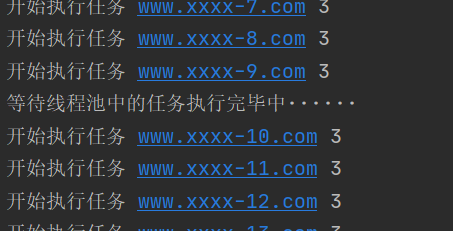﻿ 详解Python中的多线程_python_脚本之家
python# 详解Python中的多线程

## 什么是多线程：

进程：正在运行的程序，QQ 360 ......

每个线程都有自己需要运行的内容，而这些内容可以称为线程要执行的任务。

开启多线程是为了同时运行多部分代码。

好处：解决了多部分需要同时运行的问题

弊端：如果线程过多，会导致效率很低（因为程序的执行都是CPU做着随机 快速切换来完成的）

• 线程与进程的区别
• 线程共享内存，进程独立内存
• 线程启动速度块，进程启动速度慢，运行时速度没有可比性
• 同一个进程的线程间可以直接交流，两个进程想通信，必须通过一个中间代理来实现
• 创建新线程很简单，创建新进程需要对其父进程进行一次克隆
• 一个线程可以控制和操作同一线程里的其他线程，但是进程只能操作子进程

```# -*- coding:utf-8 -*-
# 线程使用的方式一
import time
# 需要多线程运行的函数
def fun(args):
print("我是线程%s" % args)
time.sleep(2)
print("线程%s运行结束" % args)
# 创建线程
start_time = time.time()
t1.start()
t2.start()
end_time = time.time()
print("两个线程一共的运行时间为：", end_time-start_time)
print("主线程结束")
"""

"""```

```# 继承式调用
import time
def __init__(self, name):
self.name = name
# 线程要运行的代码
def run(self):
print("我是线程%s" % self.name)
time.sleep(2)
print("线程%s运行结束" % self.name)
start_time = time.time()
t1.start()
t2.start()
end_time = time.time()
print("两个线程一共的运行时间为：", end_time-start_time)
print("主线程结束")
"""

"""```

## 守护线程与join方法

• 在Python多线程编程中，join方法的作用式线程同步。
• 守护线程，是为守护别人而存在的，当设置为守护线程后，被守护的主线程不存在后，守护线程也自然不存在。
• 第一种：python多线程默认情况
• Python多线程默认情况（设置线程setDaemon(False))，主线程执行完自己的任务后，就退出了，此时子线程会继续执行自己的任务，直到子线程任务结束
• 第二种：开启守护线程
• 开启线程的setDaemon(True))，设置子线程为守护线程，实现主程序结束，子程序立马全部结束功能
• 代码演示：
```# 守护线程
import time
def __init__(self, name):
self.name = name
# 线程要运行的代码
def run(self):
print("我是线程%s" % self.name)
time.sleep(2)
print("线程%s运行结束" % self.name)
start_time = time.time()
t1.setDaemon(True)
t1.start()
t2.setDaemon(True)
t2.start()
end_time = time.time()
print("两个线程一共的运行时间为：", end_time-start_time)
print("主线程结束")```
• 注意：如果要设置为守护线程，一定要在开启线程之前，将该线程设置为守护线程
• 结论：主线程结束后，无论子线程1，2是否运行完成，都结束线程，不在继续向下运行
• 第三种：加入join方法设置同步
• 当不给程序设置守护进程时，主程序将一直等待子程序全部运行完成才结束
• 代码演示：
```# join:线程同步
import time
def __init__(self, name):
self.name = name
# 线程要运行的代码
def run(self):
print("我是线程%s" % self.name)
time.sleep(3)
print("线程%s运行结束" % self.name)
start_time = time.time()
for x in range(50):
t.start()
x.join()    # 为线程开启同步
end_time = time.time()
print("50个线程一共的运行时间为：", end_time-start_time)
print("主线程结束")```

## 线程锁(互斥锁Mutex)

• 一个进程下可以启用多个线程，多个线程共享父进程的内存空间，也就意味着每个线程可以访问同一份数据，此时如果多个线程同时要修改一份数据，会出现什么状况？
• 代码演示：
```# -*- coding:utf8  -*-
import time
num = 100
def fun():
global num
print("get num:", num)
num += 1
time.sleep(1)
for x in range(200):
t.start()
x.join()
print("nun:", num)```
• 结论：运行结果可能会出现num<300的情况
• 正常来讲，这个num结果应该是300， 但在python 2.7上多运行几次，会发现，最后打印出来的num结果不总是300，为什么每次运行的结果不一样呢？ 哈，很简单，假设你有A,B两个线程，此时都 要对num 进行加1操作， 由于2个线程是并发同时运行的，所以2个线程很有可能同时拿走了num=100这个初始变量交给cpu去运算，当A线程去处完的结果是101，但此时B线程运算完的结果也是101，两个线程同时CPU运算的结果再赋值给num变量后，结果就都是101。那怎么办呢？ 很简单，每个线程在要修改公共数据时，为了避免自己在还没改完的时候别人也来修改此数据，可以给这个数据加一把锁， 这样其它线程想修改此数据时就必须等待你修改完毕并把锁释放掉后才能再访问此数据。

*注：不要在3.x上运行，不知为什么，3.x上的结果总是正确的，可能是自动加了锁

```import random
import time
num = 100
def fun():
global num
time.sleep(random.random())
lock.acquire() # 加锁
num += 1
lock.release()  # 释放锁
# 实例化锁对象
for x in range(200):
t.start()
x.join()
print("num:", num)```

## GIL VS Lock## RLock（递归锁）

```import threading, time
def run1():
lock.acquire()
print("grab the first part data")
global num
num += 1
lock.release()
return num
def run2():
lock.acquire()
print("grab the second part data")
global num2
num2 += 1
lock.release()
return num2
def run3():
lock.acquire()
res = run1()
print('--------between run1 and run2-----')
res2 = run2()
lock.release()
print(res, res2)
if __name__ == '__main__':
num, num2 = 0, 0
for i in range(3):
t.start()
else:
print(num, num2)```

```import threading
data_list = []
print("开始")
for i in range(1000000):
data_list.append(i)
print(len(data_list))
for i in range(2):
t.start()```## Semaphore（信号量）

• 互斥锁同时只允许一个线程修改数据，而Semaphore是同时允许一定数量的线程修改数据，比如厕所有三个坑，那最多只允许三个人上厕所，后面的人只能等前面的人出来才能进去。
• 代码演示：
```# -*- coding:GBK -*-
import time

sum_1 = 0
def run(i):
global sum_1
time.sleep(1)
# lock.acquire()
semaphore.acquire()
sum_1 += 1
print("线程%s来了,并修改了sum_1的值为：%s" % (i, sum_1))
semaphore.release()
# lock.release()

for x in range(10):
t.start()

pass

print("程序结束")```

## Event（事件）

• 通过Event来实现两个或多个线程间的交互，下面是一个红绿灯的例子，即起动一个线程做交通指挥灯，生成几个线程做车辆，车辆行驶按红灯停，绿灯行的规则。
• 四个常用方法

set()  # 设置标志位为 True
clear()   # 清空标志位(将标志位改为false)
is_set()  # 检测标志位，如果标志位被设置，返回True，否则返回False
wait()   # 等待标志位被设置位True程序才继续往下运行

```# -*- coding:utf-8 -*-
import time
def light():
count = 1
event.set()  # 设置标志位 True
while True:
if count <= 10:
print("现在是绿灯")
time.sleep(1)
elif count <= 15:
print("现在是红灯")
event.clear()   # 清空标志位(将标志位改为false)
time.sleep(1)
else:
count = 0
event.set()
count += 1
def car(name):
while True:
if event.is_set():
print("----------%s在起飞-------------" % name)
time.sleep(1)
else:
print("---------%s在等红灯---------------" % name)
event.wait()   # 等待标志位被设置位True程序才继续往下运行
light_1.start()
for x in range(5):
car_1.start()```

Queue（队列）

```queue.Queue(maxsize=0)
#队列：先进先出  maxsize:设置队列的大小
queue.LifoQueue(maxsize=0)
##last in fisrt out  maxsize:设置队列的大小
queue.PriorityQueue(maxsize=0)
#存储数据时可设置优先级的队列,按优先级顺序（最低的先）  maxsize:设置队列的大小  ```

exception`queue.``Empty`

Exception raised when non-blocking get()(or get_nowait()) is called on a Queue object which is empty.

exception`queue.``Full`

Exception raised when non-blocking put() (or put_nowait() ) is called on a Queue object which is full.

```import queue
# 实例化队列对象
q = queue.Queue(3)
print(q.qsize())    # 获取队列内数据的长度
print(q.empty())    # 如果队列是空的，返回True，否则返回False（不可靠！）
print(q.full())     # 如果队列已满，返回True，否则返回False（不可靠！）。
"""
Queue.put(item, block=True, timeout=None)

"""
q.put(1)            # 数据“1”进入队列
q.put("nihao")      # 数据"nihao"进入队列
q.put("456ni", block=True, timeout=5)
'''

'''
# q.put_nowait(123)

'''
Queue.get(block=True, timeout=None)

就会在必要时阻塞，直到有一个项目可用。
如果'timeout'是非负数，它最多阻断'timeout'秒，如果在这段时间内没有项目可用，则引发Empty异常。

否则引发Empty异常（'timeout'被忽略了在这种情况下）。
'''
print(q.get())
print(q.get())
print(q.get())
print(q.get(block=True, timeout=2))
'''

'''
# print(q.get_nowait())```

## 生产者消费者模型

```# 生产者/消费者
import queue
import time
# 生产者
def producer(name):
count = 1
while True:
p.put("{}骨头{}".format(name, count))
print("骨头{}被{}生产".format(count, name).center(60, "*"))
count += 1
time.sleep(0.1)
# 消费者
def consumer(name):
while True:
print("{}被{}吃掉了".format(p.get(), name))
# 实例化队列对象
p = queue.Queue(10)
# 创建生产者线程
# 创建消费者线程

## 线程池

Python3中官方才正式提供线程池。

```import threading
pass
url_list = ["www.xxxx-{}.com".format(i) for i in range(30000)]
for url in url_list:
t.start()
# 这种每次都创建一个线程去操作，创建任务的太多，线程就会特别多，可能效率反倒降低了。```

```import time
from concurrent.futures import ThreadPoolExecutor  # 并行期货，线程池执行者
"""
pool.submit(函数名,参数1，参数2，参数...)
"""
print("开始执行任务", video_url, num)     # 开始执行任务 www.xxxx-299.com 3
time.sleep(1)
# 创建线程池，最多维护10个线程
# 生成300网址，并放入列表
url_list = ["www.xxxx-{}.com".format(i) for i in range(300)]
for url in url_list:
"""
在线程池中提交一个任务，线程池如果有空闲线程，则分配一个线程去执行，执行完毕后在将线程交还给线程池，
如果没有空闲线程，则等待。注意在等待时，与主线程无关，主线程依然在继续执行。
"""
print("等待线程池中的任务执行完毕中······")
print("END")``````"""线程池的回调"""
import time
import random
print("开始执行任务", video_url)
time.sleep(1)
return random.randint(0, 10)    # 将结果封装成一个Futuer对象，返回给线程池